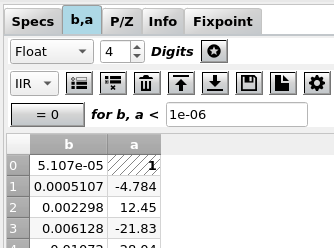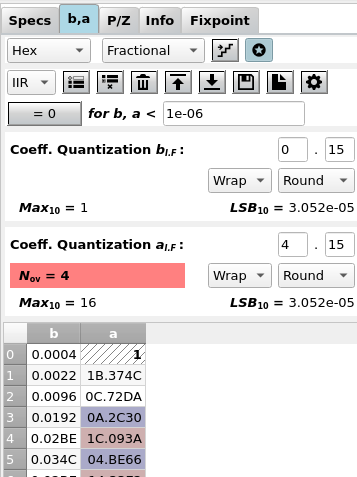# Input Coeffs¶

Fig. 7 shows a typical view of the b,a tab where you can view and edit the filter coefficients. Coefficient values are updated every time you design a new filter or update the poles / zeros.Fig. 7 Screenshot of the coefficients tab for floating point coefficients

In the top row, the display of the coefficients can be disabled as a coefficient update can be time consuming for high order filters ($$N > 100$$).

## Fixpoint¶

When the format is not float, the fixpoint options are displayed as in Fig. 8. Here, the format Binary has been set.Fig. 8 Screenshot of the coefficients tab for fixpoint formats

### Fixpoint Formats¶

Coefficients can be displayed in float format (the format returned by the filter design algorithm) with the maximum precision. This is also called “Real World Value” (RWV).

Any other format (Binary, Hex, Decimal, CSD) is a fixpoint format with a fixed number of binary places which triggers the display of further options. These formats (except for CSD) are based on the integer value i.e. by simply interpreting the bits as an integer value INT with the MSB as the sign bit

The scale between floating and fixpoint format is determined by partitioning of the word length W into integer and fractional places WI and WF. In general, W = WI + WF + 1 where the “+ 1” accounts for the sign bit.

Three kinds of partioning can be selected in a combo box:

• The integer format has no fractional bits, WF = 0 and
W = WI + 1. This is the format used by migen as well, RWV = INT
• The normalized fractional format has no integer bits, WI = 0 and
W = WF + 1.
• The general fractional format has an arbitrary number of fractional
and integer bits, W = WI + WF + 1.

In any case, scaling is determined by the number of fractional bits, $$RWV = INT \cdot 2^{-WF}$$.

$F = \frac{f}{f_S} \textrm{ or }\Omega = \frac{2\pi f}{f_S} = 2\pi F$

It is important to understand that these settings only influence the display of the coefficients, the frequency response etc. is only updated when the quantize icon (the staircase) is clicked AND afterwards the changed coefficients are saved to the dict (downwards arrow). However, when you do a fixpoint simulation or generate Verilog code from the fixpoint tab, the selected word format is used for the coefficients.

In addition to setting the position of the binary point you can select the behaviour for:

• Quantization: The very high precision of the floating point format
needs to be reduced for the fixpoint representation. Here you can select between floor (truncate the LSBs), round (classical rounding) and fix (always round to the next smallest magnitude value)
• Saturation: When the floating point number is outside the range of
the fixpoint format, either two’s complement overflow occurs (wrap) or the value is clipped to the maximum resp. minimum (“saturation”, sat)

The following shows an example of a coefficient in Q2.4 and Q0.3 format using wrap-around and truncation. It’s easy to see that for simple wrap-around logic, the sign of the result may change.

S | WI1 | WI0 * WF0 | WF1 | WF2 | WF3  :  WI = 2, WF = 4, W = 7
0 |  1  |  0  *  1  |  0  |  1  |  1   =  43 (INT) or 43/16 = 2 + 11/16 (RWV)
*
|  S  * WF0 | WF1 | WF2        :  WI = 0, WF = 3, W = 4
0  *  1  |  0  |  1         =  7 (INT) or 7/8 (RWV)